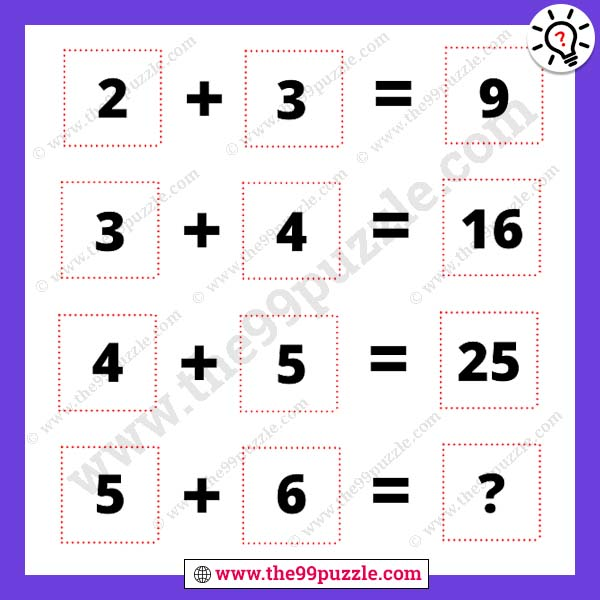# Hard and tricky math reasoning puzzle with answer – Puzz255

Are you find hard and tricky math reasoning puzzle? This math puzzle is tricky and tough to solve. Puzzles lover wants to solve this challenging and hard math question. Many math teachers fail to answer this type of reasoning question. Here this puzzle picture gives some equations. If you are a genius in mathematics you can easily find out the math logic. These math puzzles question improves logical thinking. Think logically and mathematically to find out the last equations.

2+3 = 9

3+4 = 16

4+5 = 25

5+6 = ?###### Explanation:

2+3 = (2×3)+3 = 9

3+4 = (3×4)+4 = 16

4+5 = (4×5)+5 = 25

5+6 = (5×6)+6 = 36